There are various issues if you consider the at present scenario. Some of the prominent ones are:

1. Taxes
3. Disagreements
4. Transaction costs
5. Inflation

As there are no specific numbers of problems in real world issues related to finance, separate formula for all of them is certainly not possible. So, it is always advised that whenever you trade in real world, you should keep in mind numerous problems that can crop up while doing so.

Highlighted here are certain issues.

Suppose your home base is a perfect market and you are far away from it. The first step to working with is considering a majority of market imperfections on this. There are no magic wands which can resolve those issues. It is imperative that you work those out by yourself. Many times you find the problems to be tough,but this is just because of numerous possibilities. One of the best ways to analyze the problem and search for the solution is by taking the help of numerical.

Sit back and think about a certain market situation where you can make precise assumptions. Let us in the first place think about it with perfect market in mind.Suppose there are few companies in the market who are already tax exempted. As these corporations are huge, you may think that they can easily get away. This assumption may be because of NPV formula here the better option from your side should be regarding few facts.

1. Having the idea about the direction where the perfect market can push you to
2. Judging and acknowledging the magnitude
3. Analyze the total situation and then make proper adjustment

If you follow all these steps, then you can reduce the complexities and get the accurate answer that you seek from such markets.

10.7 A. Solving an issue with the help of Taxes and Inflation

Let us assume that there is a situation where there is the presence of inflation and taxes. An important question in this scenario will be how you will be analyzing and coping with it? The answer lies exactly on the detailed steps that you should be taking to find the answer. Initially, what you need to do is start working on the answers taking some simple numbers.

Let us consider an easy amount, say \$100. With the investment of this amount, your expected return rate can be 10%. Here τ or tau will be 4%. On applying this rate on the return rate, i.e. \$10 will give you \$4. So if you calculate the total amount before tax deduction, you will be left with \$110. However, after deduction, the amount that will be with you as a nominal term will be \$106.

Here is the calculation that we have discussed above is: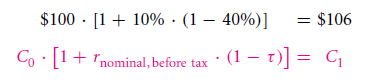Here the important aspect that you need to consider is value of that calculated \$106. This can be determined with the help of inflation introduction. To do this, we will take a round number. Suppose yearly π rate is 5%. So for the worth of \$106, purchasing power will be,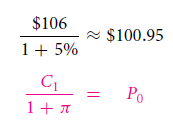After this calculation, the resultant for 3 aspects, real return rate, post inflation, and after tax is,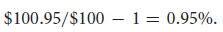This is your numerical outcome which you require converting into formula. So, the calculation will be something like: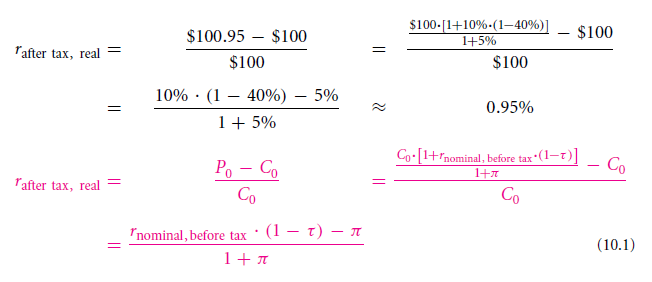This formula does have some complexities, and this is the reason why most people tend to forget it. Still, it has been depicted here in a much easier way regarding the method of its approach. With this formula, the answer to complicated questions can be easily obtained. So, you can understand now why you were suggested to use numerical in the first place and formula later.

Nominal returns and taxes on it

Now there is a question that you will find asked by a majority of investors. They seek to know how beneficial or disastrous can it be for them in case there is a hike in inflation and real return rate = 0.

To get the answer, we need to assume the constant real return rate to be 20%. In case 50% is the inflation rate, 80% will be the nominal return rate.

Computation related to it will be,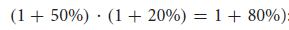With such calculation, on an investment of \$100, you will get an additional of \$80. This makes your total received amount to be \$180. On this new amount,you are required to add 40% as income tax. From the total amount, interest amount is taken to be \$80 by IRS. So, the calculation will take place over that \$80. Tax amount after computation will be \$32. After deducting \$32 from \$80, the left amount will be \$48.

Now, we will finally add this \$48 with \$100. Worth of this \$148 can be found out via this formulation,

\$148 ÷ [1 + 50%]

This will give the real value answer as \$98.67.

Here your real purchasing power with an increase is 20%. This can be only achieved with proper savings.

Now we will be calculating real purchasing power. It can be obtained via:

[(\$98.67 ÷\$100) – 1] x 100% = −1.3%

So you can see that despite real interest rate is so high, purchasing power, in this case,is way less. Claim for tax is only from gained interest. This actually is a real term loss that comes with the payment of nominal interest.

If the same circumstance is taken without inflation, your earned amount would have been \$20 with involvement of 20%real return rate. So after taxation of \$8, the real value that you would have kept for yourself would have been \$112.

Important facts to remember

Suppose nominal returns are what you get after IRS taxes and not real rate of return, and also your rate of interest for real before-taxis constant. In scenarios like this, the resultant that you will have is:

1. Taxable borrowers do get help with interest rates and higher inflation
2. Taxable savers do not get help with interest rates and higher inflation

Solve it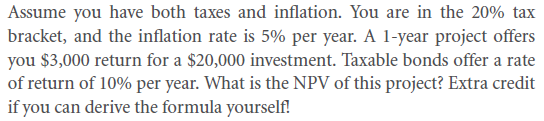2.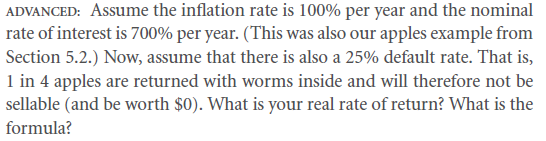3.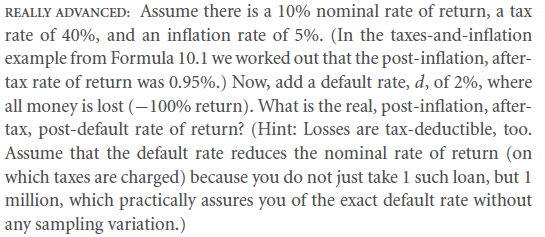4.Summary

Key Terms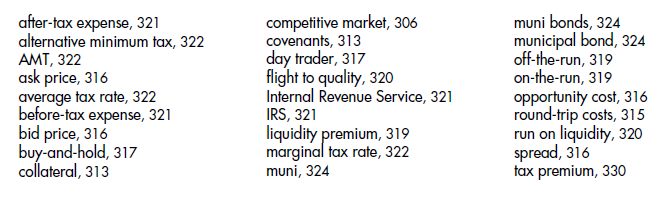Solve Now! Solutions

Problems

Links of Next Financial Accounting Topics:-### Customer Reviews

My Homework Help
Rated 5.0 out of 5 based on 510 customer reviews at